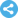American Journal of Mathematical and Computational Sciences
Manuscript Information

Fuzzy Set and Fuzzy Rough Set Concepts in Some Decision Making
American Journal of Mathematical and Computational Sciences
Vol.4 , No. 1, Publication Date: Apr. 29, 2019, Page: 19-23
354 Views Since April 29, 2019, 105 Downloads Since Apr. 29, 2019Paper in PDF (212K)Follow on

Authors

  Amarendra Baral, Department of Mathematics, Trident Academy of Technology, F2/A, Chandaka Industrial Estate, Bhubaneswar, Odisha, India.  Sambunath Behera, Department of Mathematics, BCET, Sergarh, Balasore, Odisha, India.  Purna Chandra Nayak, Department of Mathematics, Bhadrak Autonomus College, Odisha, India.

Abstract

Rapid growth in technology and its accessibility by general public produce voluminous, heterogeneous and unstructured data resulted in the emergence of new concepts. Different computational tools such as rough-set theory, fuzzy-set theory and fuzzy-rough-set that are often applied to analyze such kind of data are the focus of this chapter. Real-life data is often vague, so fuzzy logic and rough-set theory are applied to handle uncertainty and maintain consistency in the data sets. The aim of the fuzzy-rough-based method is to generate optimum variation in the range of membership functions of linguistic variables. In this paper we have discussed the definition of Fuzzy set and Fuzzy rough set. Then we have used fuzzy set and fuzzy rough set concept in some decision making of a real life problem of uncertainty. Here we have taken fuzzy set in universe X where all concepts are described. A lower and upper approximation of acoefficient of belongingness of an object x belongs to X to a fuzzy decision concept have been described. This can be done by means of a family of fuzzy concept defined on the universal set X.

Keywords

Crisp set Fuzzy Sets, Fuzzy Rough Sets, Deterministic Rough Set, Approximation, Decision Making

Reference

  A. Mrozek, Rough sets and dependency analysis among attributes in computer implementations of expert's inference models, Int. J. Man-Machine Studies 30 (1989) 457-473.  C Y Wang, Topological characterizations of generalized fuzzy rough set,” Fuzzy sets and systems, Vol. 312 pp. 109-125, 2017.  D. Dubois and H. Prade, Fuzzy Sets and Systems. Theory and Applications (Academic Press, Boston 1980).  D. Dubois and H. Prade, Twofold fuzzy sets and rough sets some issues in knowledge representation, Fuzzy Sets and Systems 23 (1987) 3-18.  D. Dubois and H. Prade, Rough sets and fuzzy rough sets, Int. J. General Systems 17 (1990) 191-209.  D. Chen. Y Yang and Z Dong. “An incremental algorith for attribute reduction with variable precision rough set” Applied soft computing Journal. Vol. 45 pp. 129-149.-2016.  F. Li and Y. Yin “θ- Lower and T-upper fuzzy rough approximationoperation on a semi group” Information sciences. An international journal. Vol. 195. pp. 241-255. 2012.  J Hu, T. Li, C. Luo, and S. Li, Incremental Fuzzy probabilistic rough sets over two universes” International journal of Approximate Reasoning. Vol. 81 pp. 28-48, 2017.  R. Slowinski, Intelligent Decision Support. Handbook of Applications and Advances of the Rough Sets Theory (Kluwer, Dordrecht, 1992).  Shen YH, Wand FX (2011) Variable precision rough set model over two universes and its properties. Soft Comput 15 (3): 557–567.  W. maa and B. Sun, Applied mathematical modelling Vol. 36 Issue 9 pp. 4539-4541 sept-2012.  Z. Pawlak, Rough sets, Int. J. Inform. Comput. ScL11 (1982) 145-172.  Z. Pawlak, Rough Sets: Theoretical Aspects of Reasoning About Data (Kluwer, Dordrecht, 1991).  Z. Pawlak, S. Wong and W. Ziarko, Rough sets: probabilistic versus deterministic approach, Int. J. Man Machine Studies 29 (1988) 81-95.  Z. Pawlak, “Rough Sets,” International Journal of Computer and Information Sciences, Vol. 11, No. 5, 1982, pp. 341-356. doi: 10.1007/BF01001956.